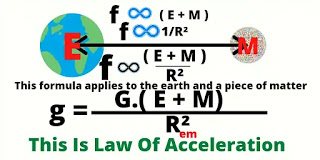# Aristotle Vs. Galileo Galileo

##Aristotle is the winner in the debate between Galileo and Aristotle

## Many will be shocked to see my heading, many will stop seeing this article but believe me today or tomorrow you will have to read this article one day. Aristotle was an early Greek thinker. He said that heavier things fall faster in proportion to their mass. But in reality we see all objects fall to the ground at the same time. It may seem that Galileo was right, but the truth is that Aristotle is more right. I'm not saying that everything Aristotle said is true, I'm just saying that heavier things fall faster in proportion to their mass, his words are true. Now let's discuss the matter a little deeper. The most advanced formula for gravitational acceleration isThis is the law of acceleration
We get from this equation
When the mass of an object is much smaller than that of the earth, we will ignore it. So,
g=G.E/R² = 985 cm/s²
When the mass of the object m is equal to the mass of the earth then
g = G.(E+E)/R² = 2.G.E/R²
Or, g = 2 × 985 = 1970cm/s²
So when an object as heavy as the mass of the earth falls on the surface of the earth, its gravitational acceleration will be 1970 cm/s².
And when the object m falls on the earth with an equal mass of the moon, then the gravitational acceleration of the earth will take place
g = G.(E+m)R²
= G.(E+E/150.74)/R²
Or, g =(1+0.006634 ).G.E/R²
g = 1 006634 × 985
= 991.5cm/s²
So if the moon falls on the surface of the earth, its gravitational acceleration will be 991.5 cm/s²
Similarly, if an object falls on the earth's surface with an equal mass of the sun, then the gravitational acceleration of the earth will be
g = G. (E+331982E)R²
= ( 1+ 331982 ).G.E/R²
= 331983 × 985 cm/s²
= 327003255 cm/s²
= 3,270 km/s²
So if the sun falls on the surface of the earth, its gravitational acceleration will be 3,270 km/s².